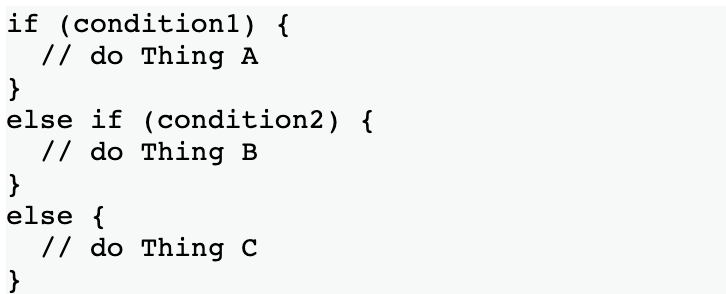top of page
Search
• Puriphico

# control structures in arduino programs (if, else, for, while, and more)

Pictured below is the general framework for the usage of control structures, which use conditions to determine the flow and order of operations in the program:While Loops:

• This function will loop continuously until the expression inside the parentheses becomes false

• If nothing changes the variable, the loop will never end; for this reason, in practice, you would not evaluate the truth of a constant, but rather the state of a sensor or the value of a variable.

The syntax for a While loop is as follows:

--------

while (condition) {

// statements;

}

--------

Here is an example of a while loop in practice. In this instance, 'var' is a variable initially set to the value 0; however, as the loop continues, a compound operator is used with '++' to increment the value of var by 1 each time the loop runs. Thus, when var is no longer less than 200, the condition is no longer true and the loop will stop executing the operations within the braces.Do...while Loops:

• Do...while loops are effectively the same as While loops, with the exception that the condition is tested at the end of the loop’s first completion. Said another way, the 'Do' syntax instructs the loop to run at least one time, and then once the first loop's first cycle has been completed, the program evaluates the truth of the condition in the while statement that follows.

Here is an example of a Do..while loop in practice. In this example, the integer 'x' is initially defined as 0; in the loop, however, 'x' is specified to be a returned value from reading the sensors. Therefore, when 'x' is no longer less than 100, no more delays and readSensors() operations will occur.If Statements:

• If statements run a statement or set of statements if the condition in parentheses is evaluated to be true.

• When using If statements, it is also possible to include other If statements within the original, and so on; however, when doing this, you must be careful to align the braces properly so that no compiling error arises.

Below you can find an example of an If statement in practice. Take note that the syntax of the if statement is very similar to that of the While and the Do...while loops, where the condition falls inside the parentheses, which is followed by an opening bracket.

Else Statements:

• The If..else statement allows for greater control over the flow and operation of the code, especially if the condition in the original If statement proves to be false. This is because, assuming the condition in the if statement is false, instead of the program continuing to do what it had been doing prior to the If statement, its outcome can be altered/specified through the Else statement that follows.

• As implied above, the Else statement will only be executed when the If statement’s condition is false.

• Each test will proceed to the next one until a true test is encountered. When a true test is found, its associated block of code is run, and the program then skips to the line following the entire if/else construction. If no test proves to be true, the default else block is executed, if one is present, and sets the default behavior.

• Between and If and Else statement, an Else If statement can be used; therefore, any number of conditions can inspire different outcomes (as pictured below).

• If the computer finds a condition that works, it will execute that function and then skip the rest of the Else and If clauses.For Loops:

• Repeats a block of code enclosed in braces. An increment counter is usually used to increment and terminate the loop. For loops are useful for any repetitive operation, especially when you want to specify the number of times the operation is executed. Below is an example syntax of a For loop:

In the below syntax, 'initialization' of the variable occurs only once (ie declaring the value of the variable), 'condition' refers to the condition that is tested each time the loop is run, and 'increment' refers to the degree at which the variable's value changes. When the condition becomes false, the loop ends.

--------

for (initialization; condition; increment) {

// statement(s);

}

--------

Here is an image of a For loop in one of my programs (note: I++ is a compound operator that increments the value of the variable, in this case, i, by 1):